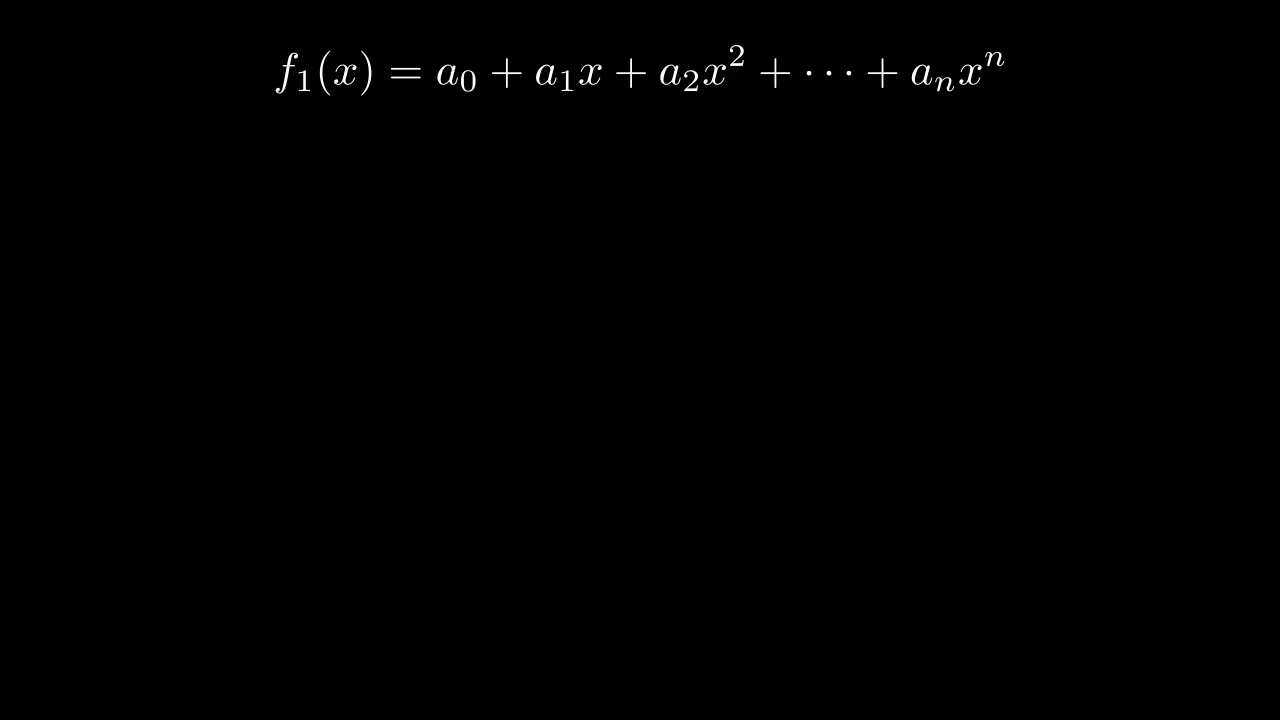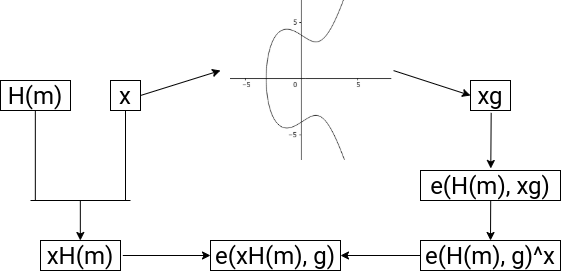# Distributed Key Generation (DKG)

## Overview

Distributed Key Generation aims to solve the problem of getting n parties able to cryptographically sign and verify signatures in the presence of some number t attackers in a decentralized network. To do so, this algorithm generates a public key, and a secret key of which no single party knows, but has some share of.

## Generating Contributions

Assume that there are n players P_1, P_2, …, P_n, and that the system should be t-secure - that is, all honest players will succeed in carrying out the algorithm in the case of at most t corrupted parties.

Each player randomly generates a secret polynomial P(x) of degree t, whose constant coefficient is their secret key. Note that one chooses degree t, since t + 1 points are required to reconstruct P. The goal here is to get other parties to agree that a player has a valid secret key, in a way that reconstructing our secret key is impossible. To do so, the player broadcasts a public polynomial P_g(x), which is generated by mapping P(x)’s coefficients to points on an elliptic curve (for generator g and coefficient x, use xg). Then, they compute the secret key contribution P(j) and send it to player j for j = 1, …, n.

## Verification and Complaints

Each player maps the outputs they receive onto points on the elliptic curve, and checks it against the corresponding public polynomial’s output. In this way, it’s verified that the player has generated a valid polynomial/output pair without exposing the polynomial itself.

If a player is unable to send their output to a recipient within a given time, or the recipient computes the output to be invalid, the recipient makes a complaint. Typically in DKG, the justification process for the complaint may involve having the output in question broadcasted to all players to verify for themselves. In SKALE’s implementation however, you use SKALE Manager which lives on the Ethereum mainnet to compute/verify each output being complained about and slash accordingly, and restart the DKG process. This has the added benefit of giving economic incentive to act honestly, and is necessary because unlike traditional DKG, there is a need to identify and replace all malicious players.

## Computing the public/private keys

Summing the public polynomials of all members results in the group’s public polynomial, whose constant coefficient is the public key; similarly, the sum of all secret polynomials results in the group’s secret polynomial. If each player sums the secret key contributions they received, they get an output of the sum of all player’s secret polynomials - or, an output of the group’s secret polynomial. This is their secret key share.

Note that the group’s secret key is created by the sum of all players’ polynomial’s constant values, and that given enough secret key shares (t + 1), one can reconstruct the the group’s secret key by recreating the group’s private polynomial by way of interpolation.

The following is a visual representation of the entire process made with Manim.## BLS Signatures

### Theory

BLS relies on the existence of an efficient bilinear pairing e: G x G → G_T for some elliptic curve G and finite field G_T, where the discrete log problem is hard on G, and whose details are technical and are therefore left out. The bilinearity condition provides that e(ag, bg) = e(g, g)^{ab}.

Note that through this mapping, one can solve the decisional Diffie-Hellman problem – given xg, yg, and zg for generator g, check that xy = z. Using this pairing, you can write e(xg, yg) = e(g, g)^{xy} = e(g, xyg), which you can compare with e(g, zg). However, finding the actual values of x, y, and z are hard due to the discrete log problem.

### Signatures and Properties

One can take advantage of the pairing’s properties to generate fast, compact, and aggregatable signatures.Firstly, the verification and signing process is very straightforward. Given a private key x and public key xg, suppose we wish to generate a signature for message m. First, we generate the hash of the message H(m) which is on the curve G, and publish xH(m). Then, verification is of the form e(xH(m), g) = e(H(m), xg), which is true by the bilinearity condition.

Now suppose you wish to verify multiple signatures for a single message. By the properties of this mapping, you know that e(A, B + C) = e(A, B) * e(A, C). Using this property, you can efficiently verify all by first obtaining the sum of the signatures S, then verifying that e(g, S) = the product of all (e(H(m_i), x_ig)). When taking this into account with the fact that BLS signatures are very short (roughly half the bits of DSA or ECDSA for similar security), BLS signatures for multiple parties save a lot of storage and computation by only needing to store and verify one value.

### Significance with DKG

Pairing DKG and BLS has a lot of nice properties. First of all, through DKG, you actually able to generate and distribute usable BLS keys. Secondly, the way DKG’s distributed secret shares and BLS’s signing algorithm interact is efficient.

Suppose you run DKG, resulting in the group’s secret polynomial being f(x), and secret key shares thus being f(i) for i . Note that you wish to keep f(i) values secret, otherwise, f(x) can be created via polynomial interpretation. Now, you generate signatures H(m)^f(i), and if you attain at least t signatures, you can run polynomial interpolation in the exponent to gain H(m)^f(0). In this way, you have generated a signature that’s equivalent to a signature generated by the group’s private key - as if it was signed by f(0) in the first place - in an efficient manner and without having exposed any secret key shares.

### Polynomial Interpolation

There are several methods of executing polynomial interpolation in the exponent. In our purposes, we choose to implement an O(t^2) algorithm, since it’s actually faster than otherwise more efficient algorithms in SKALE’s use case with smaller numbers.

To do so, one uses Lagrange polynomials. The key idea is that given points (i, y_i) for i from 1 to t, one wishes to express the final polynomial p(x) as the sum of y_i * L_i(x), where one sets up the Lagrange polynomial L_i(x) to output 1 if its input is i, and 0 if its input is j from 1 to t for j not equal to i. In this way, p(x) is a polynomial that hits all of the desired points.

Mathematically, let L_i(x) be

Here, only required to compute L_i(0), and to extrapolate p(0), raise it to the power; that is,

for secret shares s_k and group secret s = p(0).

## Known Attacks

### Joint-Feldman Protocol / Output Manipulation

In the SKALE DKG implementation, the Joint-Feldman protocol is used. In its traditional form, this protocol is vulnerable to an attack in which one malicious player purposefully generates outputs which receive complaints, which a secondary malicious player can then use the broadcasted information to generate their polynomial in such a way that biases the final result’s random distribution. However, since this implementation verifies complaints through SKALE Manager, there is no such issue since no information is exposed.

Furthermore, because of SKALE’s well-formed check for points on the elliptic curve doesn’t check that points are actually of the desired subgroup, there exists another attack in theory which involves the addition of elements of a different subgroup to submitted secret key shares. In this way, there is a chance with t - 1 malicious players that the generated public key is unusable. Again, however, SKALE’s use case makes this attack unfeasible, since the smallest subgroup of the elliptic curve in use is of order 10069, and SKALE Network has much less players than that order, which makes the attack impossible.

Thus, the Joint-Feldman protocol makes sense for SKALE Network since it’s more lightweight than otherwise more secure DKG algorithms.

### Front-Running Attack

SKALE Manager was previously vulnerable to a front-running attack for which a malicious node would not send any data, then at the moment the receiver rightfully complains about a lack of data, the node would front-run the data. At this point, SKALE Manager would see the receiver’s complaint as incorrect, and therefore slash the honest node. This has been resolved by adding strict time slots for broadcasting and complaining, and future threshold encryption will add further protection.